Next: Combined Linear Congruential Generators Up: Techniques for Generating Random Previous: Techniques for Generating Random

## Linear Congruential Method

• The linear congruential method produces a sequence of integers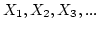between zero and m-1 according to the following recursive relationship: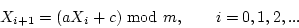• The initial value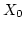is called the seed;
• a is called the constant multiplier;
• c is the increment
• m is the modulus
The selection of a, c, m anddrastically affects the statistical properties such as mean and variance, and the cycle length.

• When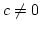, the form is called the mixed congruential method; When c = 0, the form is known as the multiplicative congruential method.

• Example 8.1 on page 292

• Issues to consider:
• The numbers generated from the example can only assume values from the set I = {0, 1/m, 2/m, ..., (m-1)/m}. If m is very large, it is of less problem. Values ofand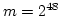are in common use.
• To achieve maximum density for a given range, proper choice of a, c, m andis very important. Maximal period can be achieved by some proven selection of these values.
• For m a power of 2, i.e.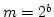, and, the longest possible period is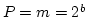, when c is relatively prime to m and a = 1 + 4 k where k is an integer.
• For m a power of 2, i.e., and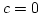, the longest possible period is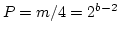, whenis odd and the multiplier, a is given by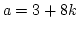or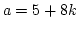where k is an integer.
• For m a prime number and c = 0, the longest possible period is P = m - 1 when a satisfies the property that the smallest k such that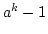is divisible by m is k = m - 1.

For example, we choose m = 7 and a = 3, the above conditions satisfy. Here k has to be 6.

• when k = 6,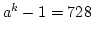which is divisible by m
• when k = 5,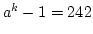which is not divisible by m
• when k = 4,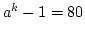which is not divisible by m
• when k = 3,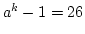which is not divisible by m
Of course, the longest possible period here is 6, which is of no practical use. But the example shows how the conditions can be checked.

• Examples 8.2, 8.3 and 8.4 on page 294 and page 295.Next: Combined Linear Congruential Generators Up: Techniques for Generating Random Previous: Techniques for Generating Random
Meng Xiannong 2002-10-18